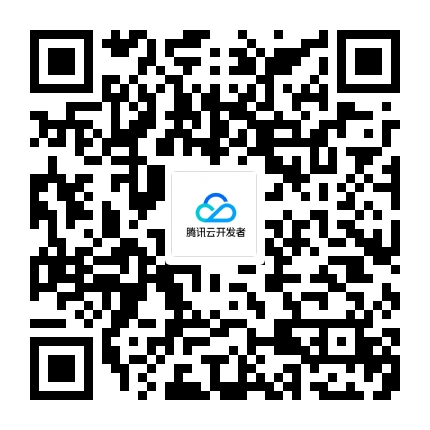TVP

# 陶泽昱Excel应用技巧大全第25期：数据筛选技术

=SUMIF(D3:D17,J3,H3:H17)

F6提取唯一记录公式：

{=INDEX(B:B,MATCH(0,COUNTIF(F\$5:F5,\$B\$2:\$B\$14)+(\$A\$2:\$A\$14\$F\$3)*(\$A\$2:\$A\$14””),0)+1)&””}

{=INDEX(B:B,SMALL(IF((A\$2:A\$13=F\$3)*(MATCH(A\$2:A\$13&B\$2:B\$13,A\$2:A\$13&B\$2:B\$13,0)=ROW(A\$2:A\$13)-1),ROW(A\$2:A\$13),4^8),ROW(A1)))&””}

{=SUM((\$A\$2:\$A\$13=\$F\$3)/COUNTIFS(\$A\$2:\$A\$13,\$A\$2:\$A\$13,\$B\$2:\$B\$13,\$B\$2:\$B\$13))}

{=INDIRECT(TEXT(MIN(IF((COUNTIF(K\$1:K1,\$A\$2:\$H\$6)=0)*(MOD(COLUMN(\$A\$1:\$H\$1),3)=2)*(\$A\$2:\$H\$6””),ROW(\$A\$2:\$H\$6)*1000+COLUMN(\$A\$2:\$H\$6),65536256)),”R0C00”),)&””}

（1）过滤条件公式段：(COUNTIF(K\$1:K1,\$A\$2:\$H\$6)=0)是去除重复数据的重点公式；(MOD(COLUMN(\$A\$1:\$H\$1),3)=2)公式段主要是为了过滤其他非商家名称数据列，目的是通过K列的自身筛选去除已经列表的商家名称。

（2）IF（过滤条件，ROW(\$A\$2:\$H\$6)*1000+COLUMN(\$A\$2:\$H\$6),65536256）公式中的65536256主要是用于容错处理（为了兼容Excel 2003的用户），通过INDIRECT(“R65536c256”,0)&””，则固定引用整个工作表（基于Excel 2003系统）的最后一个单元格，保证列表外的多余数据均显示为空白，从而省略了常规的错误判断。

（3）而INDERECT(TEXT(MIN公式段,”R0C000”),0)的用法则是一种应用技巧，它能够将行列号组合序号快速转换为单元格引用，从而返回真正的商家列表。

• 发表于:
• 原文链接https://kuaibao.qq.com/s/20180814A1TJ5T00?refer=cp_1026
• 腾讯「腾讯云开发者社区」是腾讯内容开放平台帐号（企鹅号）传播渠道之一，根据《腾讯内容开放平台服务协议》转载发布内容。
• 如有侵权，请联系 cloudcommunity@tencent.com 删除。

2023-02-04

2023-02-04

2023-02-04

2023-02-04

2023-02-04

## 关注

10元无门槛代金券